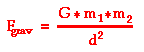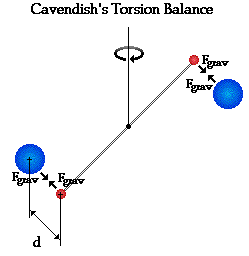Circular Motion and Satellite Motion - Lesson 3 - Universal Gravitation

# Cavendish and the Value of G

Isaac Newton's law of universal gravitation proposed that the gravitational attraction between any two objects is directly proportional to the product of their masses and inversely proportional to the square of the distance between their centers. In equation form, this is often expressed as follows:The constant of proportionality in this equation is G - the universal gravitation constant. The value of G was not experimentally determined until nearly a century later (1798) by Lord Henry Cavendish using a torsion balance.

Cavendish's apparatus for experimentally determining the value of G involved a light, rigid rod about 2-feet long. Two small lead spheres were attached to the ends of the rod and the rod was suspended by a thin wire. When the rod becomes twisted, the torsion of the wire begins to exert a torsional force that is proportional to the angle of rotation of the rod. The more twist of the wire, the more the system pushes backwards to restore itself towards the original position. Cavendish had calibrated his instrument to determine the relationship between the angle of rotation and the amount of torsional force. A diagram of the apparatus is shown below.Cavendish then brought two large lead spheres near the smaller spheres attached to the rod. Since all masses attract, the large spheres exerted a gravitational force upon the smaller spheres and twisted the rod a measurable amount. Once the torsional force balanced the gravitational force, the rod and spheres came to rest and Cavendish was able to determine the gravitational force of attraction between the masses. By measuring m1, m2, d and Fgrav, the value of G could be determined. Cavendish's measurements resulted in an experimentally determined value of 6.75 x 10-11 N m2/kg2. Today, the currently accepted value is 6.67259 x 10-11 N m2/kg2.

The value of G is an extremely small numerical value. Its smallness accounts for the fact that the force of gravitational attraction is only appreciable for objects with large mass. While two students will indeed exert gravitational forces upon each other, these forces are too small to be noticeable. Yet if one of the students is replaced with a planet, then the gravitational force between the other student and the planet becomes noticeable.

### We Would Like to Suggest ...Sometimes it isn't enough to just read about it. You have to interact with it! And that's exactly what you do when you use one of The Physics Classroom's Interactives. We would like to suggest that you combine the reading of this page with the use of our Gravitation Interactive. You can find it in the Physics Interactives section of our website. The Gravitation Interactive allows a learner to interactively explore the inverse square law of gravitation.